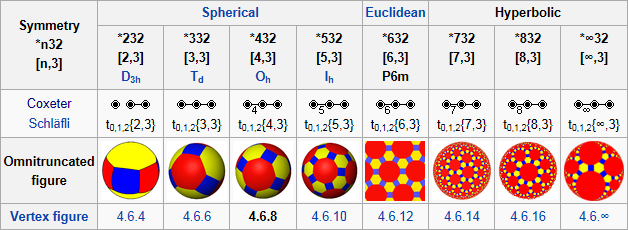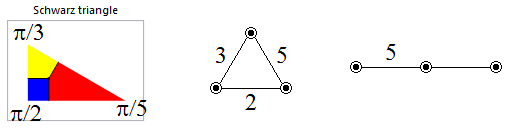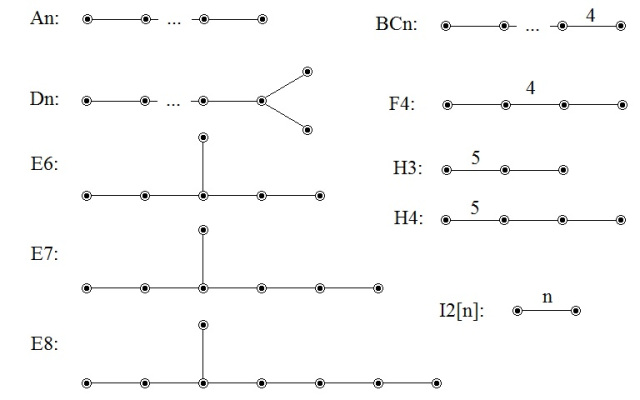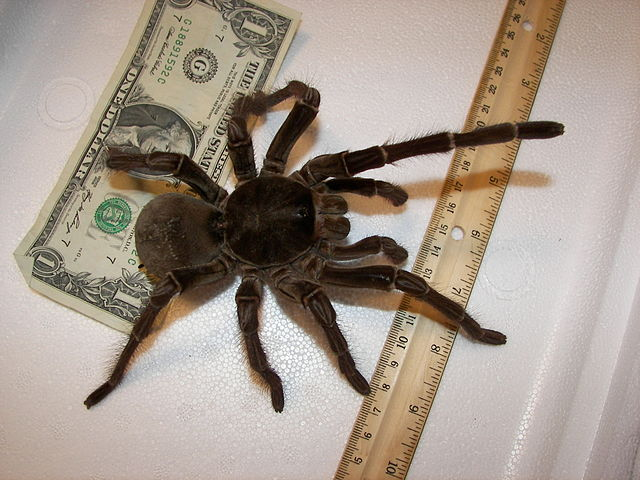# Coxeter groups and beyond

Some polyhedra have lots of symmetries. For example, consider the omnitruncated dodecahedron:The interactive demonstration constructs it kaleidoscopically, by reflecting a fundamental region (the Schwarz triangle) in three mirrors. The same fundamental region, however, can result in lots of different polyhedra and tilings of Euclidean and hyperbolic space. For example, consider the following series:These all share the same fundamental region, but with different reflection groups. To specify the omnitruncated dodecahedron, we need to include another piece of information: the angles between the mirrors. On the left of the following image is an annotated Schwarz triangle, giving the angles of 90°, 60° and 36° (these sum to more than 180°, since it is on the surface of a sphere).If we want to describe tessellations of three-dimensional space (including polychora — four-dimensional polytopes — which can be regarded as tilings of the 3-sphere), we’ll need to have a Schwarz tetrahedron instead of a Schwarz triangle.

The second diagram is the dual of the Schwarz simplex, where each node corresponds to a mirror and each edge gives the reciprocal of the angle between the mirrors. The third diagram is a simplified version, where missing edges represent ‘2’ and unlabelled edges represent ‘3’. This is called a Coxeter-Dynkin diagram.

If we have a Coxeter-Dynkin diagram with n nodes, we can construct the symmetry group as follows:

• Start with the free group on n generators, one for each node;
• Quotient out by x² = 1 for each generator x, since these generators are reflections;
• Quotient out by (xy)^n = 1 if x and y are joined by an edge labelled n. If two nodes are not connected, we have n = 2, thus xyxy = 1. Since the generators are self-inverse, xyxy is the commutator of x and y. In other words, orthogonal mirrors correspond to reflections that commute with each other.

This gives a presentation of the group with ½(n² + n) relations on n generators.

### Finite Coxeter groups

The finite reflection groups have been completely classified, and correspond to one of the following diagrams:H3 is the familiar icosahedral symmetry, and H4 is the four-dimensional analogue. I2[n] is simply the reflection group of the regular n-gon (and therefore is isomorphic to the dihedral group D_2n). An and BCn are the symmetries of the regular simplex and hypercube, respectively. Dn is an index-2 subgroup of BCn corresponding to the symmetries that fix a 2-colouring of the vertices of the hypercube. F4 is the Coxeter group of the 24-cell (a special self-dual polytope in four dimensions).

This leaves three more exceptional cases, E6, E7 and E8. They’re fascinating objects, and really worth discussing, but I don’t really have time to discuss them at the moment. However, because it’s the most beautiful, I’ll use E8 as an example for presenting a Coxeter group:### The spider relation

Whilst E6, E7 and E8 are very interesting Coxeter groups, their exceptionality is rivalled only by the sporadic groups. Rather amazingly, some of the bigger sporadic groups can be obtained as quotients of infinite Coxeter groups. For example, start with the Coxeter group of the following diagram:This is a huge infinite hyperbolic Coxeter group, G555. Nevertheless, a single extra relation, called the spider relation, causes it to collapse into a finite group, Y555:

• (a b1 c1 a b2 c2 a b3 c3)^10 = 1

As an aid to memory, here is a completely gratuitous photograph of a large spider:Image courtesy of someone called John, shared under the Creative Commons licence.

The relevant nodes are highlighted in red in the Coxeter-Dynkin diagram. Ivanov and Norton proved a conjecture by Conway that Y555 is, in fact, the wreath product of the Monster group with the cyclic group C2. Consequently, it is a group of order 2m², where m is the (very large!) order of the Monster group.

This group, the bimonster, can also be constructed as Y554, Y544 or Y444. Similarly, the groups Y553, Y543 and Y443 are isomorphic to the direct product of the Monster group and C2. Hence, we have a presentation of the Monster group on 12 generators and 80 relations (78 for the Coxeter group, together with 1 spider relation and the relation Z = 1, where Z is the unique order-2 element in the centre of the group).

This entry was posted in Uncategorized. Bookmark the permalink.